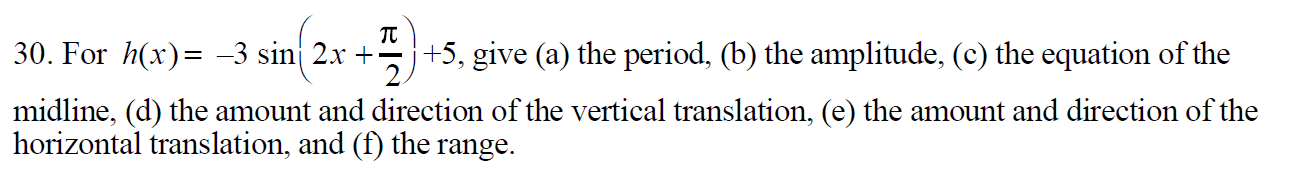TT+5, give (a) the period, (b) the amplitude, (c) the equation of the2.30. For x)-3 sin 2x +midline, (d) the amount and direction of the vertical translation, (e) the amount and direction of thehorizontal translation, and (f) the range

Questionhelp_outlineImage TranscriptioncloseTT +5, give (a) the period, (b) the amplitude, (c) the equation of the 2. 30. For x) -3 sin 2x + midline, (d) the amount and direction of the vertical translation, (e) the amount and direction of the horizontal translation, and (f) the range fullscreen
Step 1

You have asked a question with multiple sub parts. I will address the first three sub parts of the question. Please post the balance sub parts as a separate question.

A standard Simple Harmonic Motion (SHM) is given by: ± A sin (Bx + C)

For such a standard SHM, A = amplitude; Time period = 2 π / B

Our equation is: h(x) = -3 sin (2x +  π /2) + 5

A = 3; B = 2

Step 2

Part (a)

Time period = 2π / B = 2π / 2 =  π

Step 3

Part (b)

The amplitu...

Want to see the full answer?

See Solution

Want to see this answer and more?

Our solutions are written by experts, many with advanced degrees, and available 24/7

See Solution
Tagged in

Functions